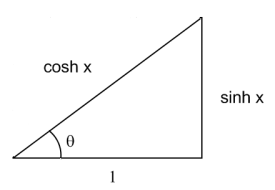# Inverse Mercator projection

In my earlier post on the Mercator projection, I derived the function h(φ) that maps latitude on the Earth to vertical height on a map. The inverse of this function turns out to hold a few surprises.

The height y corresponding to a positive latitude φ is given by

h(φ) = log( sec(φ) + tan(φ) ).

The inverse function, h-1(y) = φ gives the latitude as a function of height. This function is called the “Gudermannian” after Christoph Gudermann and is abbreviated gd(y). Gudermann was the student of one famous mathematician, Karl Friedrich Gauss, and the teacher of another famous mathematician, Karl Weierstrass.

The Gudermannian function gd(y) can be reduced to familiar functions:

gd(y) = arctan( sinh(y) ) = 2 arctan( ey ) – π/2.

That doesn’t look very promising. But here’s the interesting part: the function gd forms a bridge between hyperbolic trig functions and ordinary trig functions.

sin( gd(x) ) = tanh(x)
tan( gd(x) ) = sinh(x)
cos( gd(x) ) = sech(x)
sec( gd(x) ) = cosh(x)
csc( gd(x) ) = coth(x)
cot( gd(x) ) = csch(x)

By definition, gd(x) is an angle θ whose tangent is sinh(x).In the figure, tan(θ) = sinh(x). Since cosh2(x) – sinh2(x) = 1, the hypotenuse of the triangle is cosh(x). The identities above follow directly from the figure. For example, sin(θ) = sinh(x) / cosh(x) = tanh(x).

Finally, it is easy to show that gd is the inverse of the Mercator scale function h:

h( gd(x) ) = log( sec( gd(x) ) + tan( gd(x) ) ) = log( cosh(x) + sinh(x) ) = log( ex ) = x.